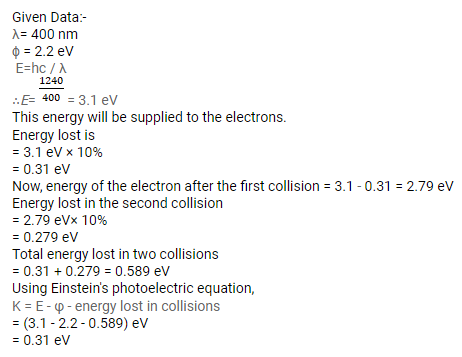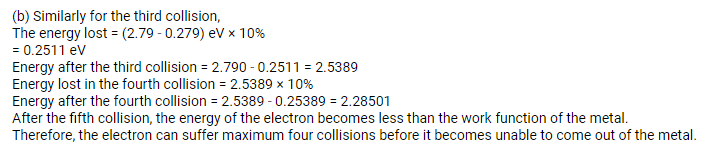# A light beam of wavelength 400 nm is incident on a metal plate of work function 2.2 eV.

Question:

A light beam of wavelength $400 \mathrm{~nm}$ is incident on a metal plate of work function $2.2 \mathrm{eV}$.

(a) A particular electron absorbs a photon and makes two collisions before coming out of the metal. Assuming that $10 \%$ of the extra energy is lost to the metal in each collision, find the kinetic energy of this electron as it comes out of the metal.

(b) Under the same assumptions, find the maximum number of collisions the electron can suffer before it becomes unable to come out of the metal.

Solution: